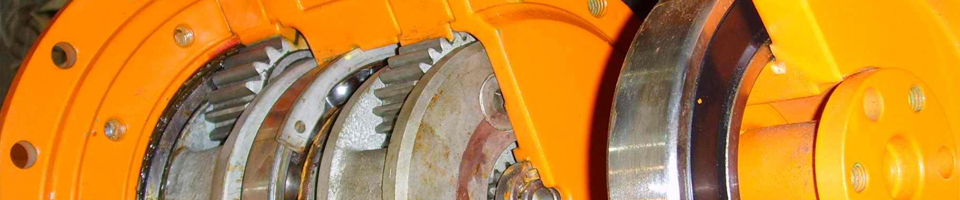# 圆柱齿轮减速机E-mail：28344966@qq.com

#### 两级齿轮减速机设计说明书

1. 传动装置总体设计方案
2. 电动机的选择
3. 确定传动装置的总传动比和分配传动比
4. 计算传动装置的运动和动力参数
5. 设计V带和带轮
6. 齿轮的设计
7. 滚动轴承和传动轴的设计
8. 键联接设计
9. 箱体结构设计
10. 润滑密封设计
11. 联轴器设计

1.传动装置总体设计方案:

1. 组成：传动装置由电机、减速器、工作机组成。
2. 特点：齿轮相对于轴承不对称分布，故沿轴向载荷分布不均匀，

3. 确定传动方案：考虑到电机转速高，传动功率大，将V带设置在高速级。

图一:(传动装置总体设计图)

＝0.96× × ×0.97×0.96＝0.759；

2.电动机的选择

P
kw 电动机转速

N 参考价格

同步转速 满载转速    总传动比 V带传动 减速器
1 Y112M-4 4 1500 1440 470 230 16.15 2.3 7.02

L×（AC/2+AD）×HD 底脚安装尺寸A×B 地脚螺栓孔直径K 轴伸尺寸D×E 装键部位尺寸F×GD
132 515× 345× 315 216 ×178 12 36× 80 10 ×41

3.确定传动装置的总传动比和分配传动比
（1）       总传动比

（2）       分配传动装置传动比
＝ ×

4.计算传动装置的运动和动力参数
（1）　各轴转速
＝ ＝1440/2.3＝626.09r/min
＝ ＝626.09/3.24＝193.24r/min
＝ / ＝193.24/2.33=82.93 r/min
= =82.93 r/min
（2）　各轴输入功率
＝ × ＝3.25×0.96＝3.12kW
＝ ×η2× ＝3.12×0.98×0.95＝2.90kW
＝ ×η2× ＝2.97×0.98×0.95＝2.70kW
＝ ×η2×η4=2.77×0.98×0.97＝2.57kW

＝ ×0.98=3.06 kW
＝ ×0.98=2.84 kW
＝ ×0.98=2.65kW
＝ ×0.98=2.52 kW
（3） 各轴输入转矩
= × ×   N·m

＝ × × × =47.58×3.24×0.98×0.95=143.53 N·m
＝ × × × =143.53×2.33×0.98×0.95=311.35N·m
= × × =311.35×0.95×0.97=286.91 N·m

＝ ×0.98=140.66 N·m
＝ ×0.98=305.12N·m
＝ ×0.98=281.17 N·m

1轴 3.12 3.06 47.58 46.63 626.09
2轴 2.90 2.84 143.53 140.66 193.24
3轴 2.70 2.65 311.35 305.12 82.93
4轴 2.57 2.52 286.91 281.17 82.93

6.齿轮的设计
（一）高速级齿轮传动的设计计算
１． 齿轮材料，热处理及精度

（1）       齿轮材料及热处理
① 材料：高速级小齿轮选用 钢调质，齿面硬度为小齿轮 280HBS 取小齿齿数 =24

② 齿轮精度

２．初步设计齿轮传动的主要尺寸

①试选 =1.6

②由课本 公式10-13计算应力值环数
N =60n j =60×626.09×1×（2×8×300×8）
=1.4425×10 h
N = =4.45×10 h #(3.25为齿数比,即3.25= )
③查课本 10-19图得：K =0.93   K =0.96
④齿轮的疲劳强度极限

[ ] = =0.93×550=511.5
[ ] = =0.96×450=432

⑤查课本由 表10-6得： =189.8MP
由 表10-7得: =1
T=95.5×10 × =95.5×10 ×3.19/626.09
=4.86×10 N.m
3.设计计算
①小齿轮的分度圆直径d
=
②计算圆周速度

③计算齿宽b和模数

b= =49.53mm

=
④计算齿宽与高之比

= =11.01
⑤计算纵向重合度
=0.318 =1.903
⑥计算载荷系数K

K =   +0.23×10 ×b
=1.12+0.18(1+0.6 1) ×1+0.23×10 ×49.53=1.42

K＝K K K K =1×1.07×1.2×1.42=1.82
⑦按实际载荷系数校正所算得的分度圆直径
d =d =49.53× =51.73
⑧计算模数
=
4. 齿根弯曲疲劳强度设计

⑴   确定公式内各计算数值
①   小齿轮传递的转矩 ＝48.6kN·m
确定齿数z

Δi＝0.032％ 5％，允许
②      计算当量齿数
z ＝z /cos ＝24/ cos 14 ＝26.27
z ＝z /cos ＝78/ cos 14 ＝85.43
③       初选齿宽系数
按对称布置，由表查得 ＝1
④       初选螺旋角
初定螺旋角 ＝14
⑤       载荷系数K
K＝K K K K =1×1.07×1.2×1.35＝1.73
⑥       查取齿形系数Y 和应力校正系数Y

⑦       重合度系数Y

＝arctg（tg /cos ）＝arctg（tg20 /cos14 ）＝20.64690
＝14.07609

⑧       螺旋角系数Y

Y ＝1－ ＝0.78
⑨       计算大小齿轮的

K =0.86        K =0.93

[ ] =
[ ] =

⑵   设计计算
① 计算模数

z = =25.097 取z =25

②   几何尺寸计算

=arccos

d = =51.53
d = =166.97

B=

（二） 低速级齿轮传动的设计计算
⑴   材料：低速级小齿轮选用 钢调质，齿面硬度为小齿轮 280HBS 取小齿齿数 =30

⑵   齿轮精度

⑶ 按齿面接触强度设计
1. 确定公式内的各计算数值
①试选K =1.6
②查课本由 图10-30选取区域系数Z =2.45
③试选 ,查课本由 图10-26查得
=0.83    =0.88   =0.83+0.88=1.71

N =60×n ×j×L =60×193.24×1×(2×8×300×8)
=4.45×10
N = 1.91×10

K =0.94                   K = 0.97

[ ] = =
[ ] = =0.98×550/1=517
[ 540.5

T=95.5×10 × =95.5×10 ×2.90/193.24
=14.33×10 N.m
=65.71
2.   计算圆周速度
0.665
3.   计算齿宽
b= d =1×65.71=65.71
4.   计算齿宽与齿高之比

=65.71/5.4621=12.03
5.   计算纵向重合度
6.   计算载荷系数K
K =1.12+0.18(1+0.6 +0.23×10 ×b
=1.12+0.18(1+0.6)+ 0.23×10 ×65.71=1.4231

=1.04   K =1.35    K =K =1.2

K＝ =1×1.04×1.2×1.4231=1.776
7.   按实际载荷系数校正所算的分度圆直径
d =d =65.71×

3.   按齿根弯曲强度设计
m≥
㈠确定公式内各计算数值
（1）       计算小齿轮传递的转矩 ＝143.3kN·m
（2）       确定齿数z

Δi＝0.032％ 5％，允许
（3）       初选齿宽系数
按对称布置，由表查得 ＝1
（4）      初选螺旋角

（5）      载荷系数K
K＝K K K K =1×1.04×1.2×1.35＝1.6848
（6） 当量齿数
z ＝z /cos ＝30/ cos 12 ＝32.056
z ＝z /cos ＝70/ cos 12 ＝74.797

（7）       螺旋角系数Y

Y ＝1－ ＝0.797
（8）       计算大小齿轮的

K =0.90      K =0.93    S=1.4
[ ] =
[ ] =
计算大小齿轮的 ,并加以比较

① 计算模数

z = =27.77 取z =30
z =2.33×30=69.9     取z =70
②   初算主要尺寸

=arccos

分度圆直径
d = =61.34
d = =143.12

低速级大齿轮如上图：

V带齿轮各设计参数附表
1.各传动比
V带 高速级齿轮 低速级齿轮
2.3 3.24 2.33
2. 各轴转速n
(r/min)
(r/min) (r/min)
(r/min)
626.09 193.24 82.93 82.93
3. 各轴输入功率 P
（kw）
（kw）
（kw）
(kw)
3.12    2.90 2.70 2.57
4. 各轴输入转矩 T
(kN·m)
(kN·m) (kN·m)   (kN·m)
47.58 143.53 311.35 286.91
5. 带轮主要参数

90 224 471 1400 5

7.传动轴承和传动轴的设计
1. 传动轴承的设计
⑴. 求输出轴上的功率P ，转速 ，转矩
P =2.70KW             =82.93r/min
=311.35N．m
⑵. 求作用在齿轮上的力

=143.21

F = F
F = F tan =4348.16×0.246734=1072.84N

⑶.   初步确定轴的最小直径

⑷.   根据轴向定位的要求确定轴的各段直径和长度
① 为了满足半联轴器的要求的轴向定位要求,Ⅰ-Ⅱ轴段右端需要制出一轴肩,故取Ⅱ-Ⅲ的直径 ;左端用轴端挡圈定位,按轴端直径取挡圈直径 半联轴器与   为了保证轴端挡圈只压在半联轴器上而不压在轴端上, 故Ⅰ-Ⅱ的长度应比 略短一些,现取
② 初步选择滚动轴承.因轴承同时受有径向力和轴向力的作用,故选用单列角接触球轴承.参照工作要求并根据 ,由轴承产品目录中初步选取0基本游隙组 标准精度级的单列角接触球轴承7010C型.

D B

45 85 19 58.8 73.2 7209AC
45 85 19 60.5 70.2 7209B
45 100 25 66.0 80.0 7309B
50   80   16   59.2 70.9 7010C
50   80   16   59.2 70.9 7010AC
50   90   20   62.4 77.7 7210C

2. 从动轴的设计
对于选取的单向角接触球轴承其尺寸为的 ,故 ;而   .

③   取安装齿轮处的轴段 ;齿轮的右端与左轴承之间采用套筒定位.已知齿轮 的宽度为75mm,为了使套筒端面可靠地压紧齿轮,此轴段应略短于轮毂宽度,故取 . 齿轮的左端采用轴肩定位,轴肩高3.5,取 .轴环宽度 ,取b=8mm.
④    轴承端盖的总宽度为20mm(由减速器及轴承端盖的结构设计而定) .根据轴承端盖的装拆及便于对轴承添加润滑脂的要求,取端盖的外端面与半联轴器右端面间的距离 ,故取 .
⑤    取齿轮距箱体内壁之距离a=16 ,两圆柱齿轮间的距离c=20 .考虑到箱体的铸造误差,在确定滚动轴承位置时,应距箱体内壁一段距离 s,取s=8 ,已知滚动轴承宽度T=16 ,

5.    求轴上的载荷

(从动轴)

(中间轴)
(主动轴)

从动轴的载荷分析图:
6.     按弯曲扭转合成应力校核轴的强度

= =

〈 [ ]    此轴合理安全
7.     精确校核轴的疲劳强度.
⑴.   判断危险截面

⑵. 截面Ⅶ左侧。

= =

2.0          =1.31

=0.85
K =1+ =1.82
K =1+ （ -1）=1.26

K =1.62

取0.05

S = 25.13
S 13.71
≥S=1.5    所以它是安全的

= = K =
K =

K =2.8    K =1.62

取0.1        取0.05

S = 25.13
S 13.71
≥S=1.5    所以它是安全的
8.键的设计和计算
①选择键联接的类型和尺寸

b =20     h =12      =50
②校和键联接的强度

50-20=30
③键与轮毂键槽的接触高度
K =0.5 h =5
K =0.5 h =6

＜[ ]
＜[ ]

键2：16×36 A GB/T1096-1979

9.箱体结构的设计

1.   机体有足够的刚度

2.   考虑到机体内零件的润滑，密封散热。

3.   机体结构有良好的工艺性.

4.   对附件设计
A 视孔盖和窥视孔

B 油螺塞：

C 油标：

D 通气孔：

E 盖螺钉：

F 位销：

G 吊钩：

120（1轴）125（2轴）
150（3轴）

120（1轴）125（2轴）
150（3轴）
10. 润滑密封设计

H=30   =34

[本文观点非特殊说明，均有泰兴减速机-圆柱齿轮减速机整理原创所得，（转载必究）如要转载请保留相应的源地址，尊重他人劳动成果]